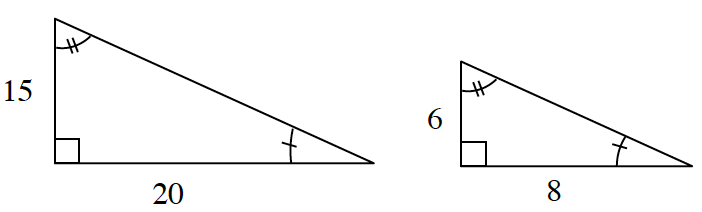### Home > CCG > Chapter 3 > Lesson 3.1.2 > Problem3-19

3-19.

The ratios Casey wrote from part (a) of problem 3-15 are common ratios between corresponding sides of the two shapes. That is, they are ratios between the matching sides of two shapes.

1. Look at the two similar shapes below. Which sides correspond? Write the common ratios using the side lengths.The sides labeled $15$ and $6$ are corresponding, as are the sides labeled $20$ and $8$.

2. Find the hypotenuse of each triangle above. Is the ratio of the hypotenuses equal to the ratios you found in part (a)?

Refer to the Math Notes box in this lesson (3.1.2) if you need help writing common ratios.

Use the Pythagorean Theorem to solve for the hypotenuse.

$15^2+20^2=c^2$

$625=c^2$

$\sqrt{625} = \sqrt{c^2}$

$c=25$
(Note: the second solution $(-25)$ is rejected for this problem.)

Use this method to find the hypotenuse of the second triangle.

Is the ratio of the hypotenuses equal to the ratios you wrote in part (a)?